Interference of Waves

3-3-99

Sections 11.11 - 11.12

Interference

Interference is what happens when two or more waves come together. Depending on how the peaks and troughs of the waves are matched up, the waves might add together or they can partially or even completely cancel each other. We'll discuss interference as it applies to sound waves, but it applies to other waves as well.

Linear superposition

The principle of linear superposition - when two or more waves come together, the result is the sum of the individual waves.

The principle of linear superposition applies to any number of waves, but to simplify matters just consider what happens when two waves come together. For example, this could be sound reaching you simultaneously from two different sources, or two pulses traveling towards each other along a string. When the waves come together, what happens? The result is that the waves are superimposed: they add together, with the amplitude at any point being the addition of the amplitudes of the individual waves at that point.

Although the waves interfere with each other when they meet, they continue traveling as if they had never encountered each other. When the waves move away from the point where they came together, in other words, their form and motion is the same as it was before they came together.

Constructive interference

Constructive interference occurs whenever waves come together so that they are in phase with each other. This means that their oscillations at a given point are in the same direction, the resulting amplitude at that point being much larger than the amplitude of an individual wave. For two waves of equal amplitude interfering constructively, the resulting amplitude is twice as large as the amplitude of an individual wave. For 100 waves of the same amplitude interfering constructively, the resulting amplitude is 100 times larger than the amplitude of an individual wave. Constructive interference, then, can produce a significant increase in amplitude.

The following diagram shows two pulses coming together, interfering constructively, and then continuing to travel as if they'd never encountered each other.Another way to think of constructive interference is in terms of peaks and troughs; when waves are interfering constructively, all the peaks line up with the peaks and the troughs line up with the troughs.

Destructive interference

Destructive interference occurs when waves come together in such a way that they completely cancel each other out. When two waves interfere destructively, they must have the same amplitude in opposite directions. When there are more than two waves interfering the situation is a little more complicated; the net result, though, is that they all combine in some way to produce zero amplitude. In general, whenever a number of waves come together the interference will not be completely constructive or completely destructive, but somewhere in between. It usually requires just the right conditions to get interference that is completely constructive or completely destructive.

The following diagram shows two pulses interfering destructively. Again, they move away from the point where they combine as if they never met each other.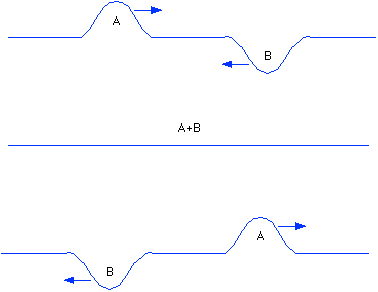Reflection of waves

This applies to both pulses and periodic waves, although it's easier to see for pulses. Consider what happens when a pulse reaches the end of its rope, so to speak. The wave will be reflected back along the rope.

If the end is fixed, the pulse will be reflected upside down (also known as a 180° phase shift).

If the end is free, the pulse comes back the same way it went out (so no phase change).

If the pulse is traveling along one rope tied to another rope, of different density, some of the energy is transmitted into the second rope and some comes back. For a pulse going from a light rope to a heavy rope, the reflection occurs as if the end is fixed. From heavy to light, the reflection is as if the end is free.

Standing waves

Moving on towards musical instruments, consider a wave travelling along a string that is fixed at one end. When the wave reaches the end, it will be reflected back, and because the end was fixed the reflection will be reversed from the original wave (also known as a 180° phase change). The reflected wave will interfere with the part of the wave still moving towards the fixed end. Typically, the interference will be neither completely constructive nor completely destructive, and nothing much useful occurs. In special cases, however, when the wavelength is matched to the length of the string, the result can be very useful indeed.

Consider one of these special cases, when the length of the string is equal to half the wavelength of the wave.

time to produce half a wavelength is t = T / 2 = 1 / 2f
in this time the wave travels at a speed v a distance L, so t = L / v
combining these gives L / v = 1 / 2f, so f = v / 2L

This frequency is known as the first harmonic, or the fundamental frequency, of the string. The second harmonic will be twice this frequency, the third three times the frequency, etc. The different harmonics are those that will occur, with various amplitudes, in stringed instruments.

String instruments and transverse standing waves

In general, the special cases (the frequencies at which standing waves occur) are given by: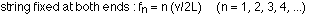The first three harmonics are shown in the following diagram: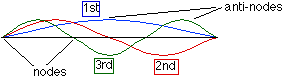When you pluck a guitar string, for example, waves at all sorts of frequencies will bounce back and forth along the string. However, the waves that are NOT at the harmonic frequencies will have reflections that do NOT constructively interfere, so you won't hear those frequencies. On the other hand, waves at the harmonic frequencies will constructively interfere, and the musical tone generated by plucking the string will be a combination of the different harmonics.

Example - a particular string has a length of 63.0 cm, a mass of 30 g, and has a tension of 87.0 N. What is the fundamental frequency of this string? What is the frequency of the fifth harmonic?

The first step is to calculate the speed of the wave (F is the tension):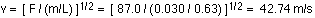The fundamental frequency is then found from the equation: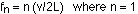So the fundamental frequency is 42.74 / (2 x 0.63) = 33.9 Hz.

The second harmonic is double that frequency, and so on, so the fifth harmonic is at a frequency of 5 x 33.9 = 169.5 Hz.